+关注继续查看

# 一、数值微分

## 1.导数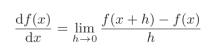python实现的代码如下：

def numerical_diff(f, x):
"""
:param f: f为x的为函数表达式
:param x: 函数f在值x处求导数
:return:  返回函数f在x处的导数值
"""
h = 1e-5 # h取一个很小的值
return (f(x+h) - f(x)) / (h)


def numerical_diff(f, x):
h = 1e-5 # h取一个很小的值
return (f(x+h) - f(x-h)) / (2 * h)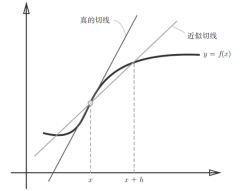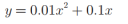def numerical_diff(f, x):
"""
:param f: f为x的为函数表达式
:param x: 函数f在值x处求导数
:return:  返回函数f在x处的导数值
"""
h = 1e-5 # h取一个很小的值
return (f(x+h) - f(x)) / (h)import numpy as np
import matplotlib.pylab as plt
def numerical_diff(f, x):
h = 1e-4  # 0.0001
return (f(x + h) - f(x - h)) / (2 * h)

def function_1(x):
return 0.01 * x ** 2 + 0.1 * x

x = np.arange(0.0, 20.0, 0.1)
y = function_1(x)
plt.xlabel("x")
plt.ylabel("f(x)")
plt.plot(x, y)
plt.show()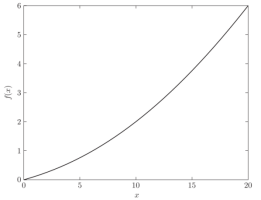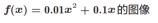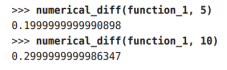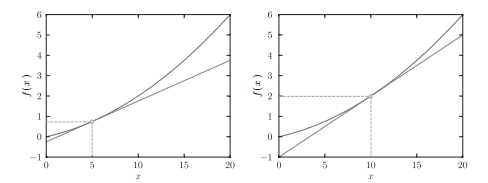## 2.偏导数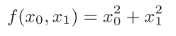def function_2(x):
# 传入的x为一个二元输出[x0,x1]
return x**2 + x**2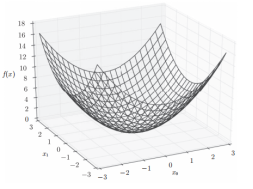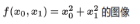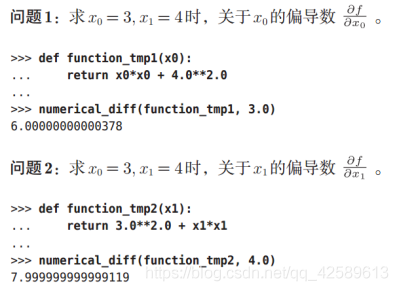## 3.梯度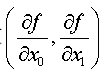import numpy as np
import matplotlib.pylab as plt

def function_2(x):
return np.sum(x ** 2)

h = 1e-4  # 0.0001

for idx in range(x.size):
# 遍历每一个自变量索引，tmp_val保存当前自变量值
tmp_val = x[idx]
x[idx] = float(tmp_val) + h
fxh1 = f(x)  # 计算f(x+h)

x[idx] = tmp_val - h
fxh2 = f(x)  # 计算f(x-h)
grad[idx] = (fxh1 - fxh2) / (2 * h)

x[idx] = tmp_val  # 将当前自变量xi值还原，继续计算后续x[i+1]的导数值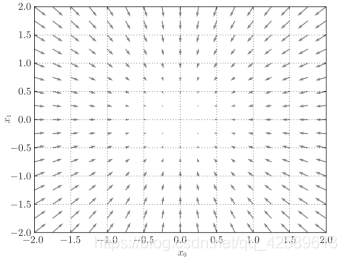# 二、梯度法

## 1.学习率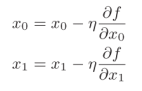η表示更新量，在神经网络的学习中，称为学习率（ learningrate）。学习率决定在一次学习中，应该学习多少，以及在多大程度上更新参数。

import numpy as np
def function_2(x):
return np.sum(x ** 2)

# 求每一个参数的梯度值
h = 1e-4  # 0.0001

for idx in range(x.size):
# 遍历每一个自变量索引，tmp_val保存当前自变量值
tmp_val = x[idx]
x[idx] = float(tmp_val) + h
fxh1 = f(x)  # 计算f(x+h)
x[idx] = tmp_val - h
fxh2 = f(x)  # 计算f(x-h)
grad[idx] = (fxh1 - fxh2) / (2 * h)
x[idx] = tmp_val  # 将当前xi值还原，继续计算后续x[i+1]的导数

# lr：学习率设为0.01
# 梯度下降的步数step_num=100
x = init_x
for i in range(step_num):
# 对每个参数进行100次梯度下降计算
return x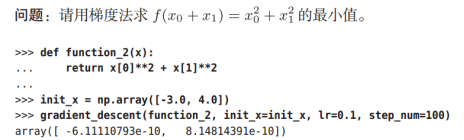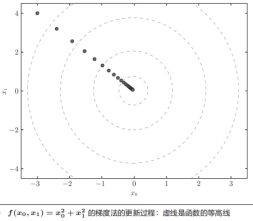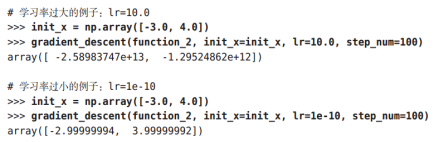## 2.神经网络的梯度计算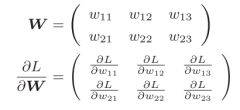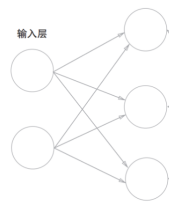import numpy as np

def softmax(x):
# softmax激活函数
if x.ndim == 2:
x = x.T
x = x - np.max(x, axis=0)
y = np.exp(x) / np.sum(np.exp(x), axis=0)
return y.T

x = x - np.max(x) # 溢出对策
return np.exp(x) / np.sum(np.exp(x))

def cross_entropy_error(y, t):
# 交叉熵误差
if y.ndim == 1:
t = t.reshape(1, t.size)
y = y.reshape(1, y.size)
# 监督数据是one-hot-vector的情况下，转换为正确解标签的索引
if t.size == y.size:
t = t.argmax(axis=1)
batch_size = y.shape
return -np.sum(np.log(y[np.arange(batch_size), t] + 1e-7)) / batch_size

# 计算所有参数x的梯度
h = 1e-4  # 0.0001
while not it.finished:
idx = it.multi_index
tmp_val = x[idx]
x[idx] = float(tmp_val) + h
fxh1 = f(x)  # f(x+h)

x[idx] = tmp_val - h
fxh2 = f(x)  # f(x-h)
grad[idx] = (fxh1 - fxh2) / (2 * h)

x[idx] = tmp_val  # 还原值
it.iternext()

class simpleNet:
def __init__(self):
# 2 X 3的单层神经网络为例求梯度
self.W = np.random.randn(2,3)

def predict(self, x):
# 计算预测值
return np.dot(x, self.W)

def loss(self, x, t):
z = self.predict(x)
# 使用sofmax为激活函数
y = softmax(z)
# 使用交叉熵误差
loss = cross_entropy_error(y, t)
return loss

x = np.array([0.6, 0.9])
t = np.array([0, 0, 1])

net = simpleNet()
# 损失函数
f = lambda w: net.loss(x, t)
# 损失函数的梯度
print(dW)


# 总结

1.介绍了基本的数值微分知识：导致、偏导数及梯度；
2.神经网络需要选择合适的学习率才能够更好的进行训练；
3.以单层神经网络为例，介绍了神经网络梯度的计算方式。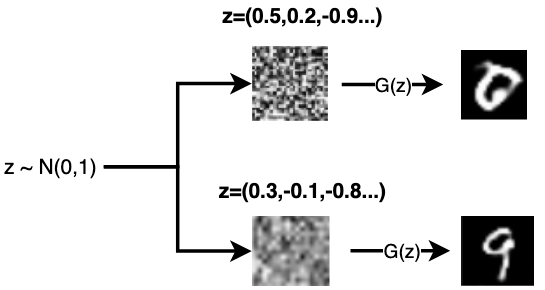18 0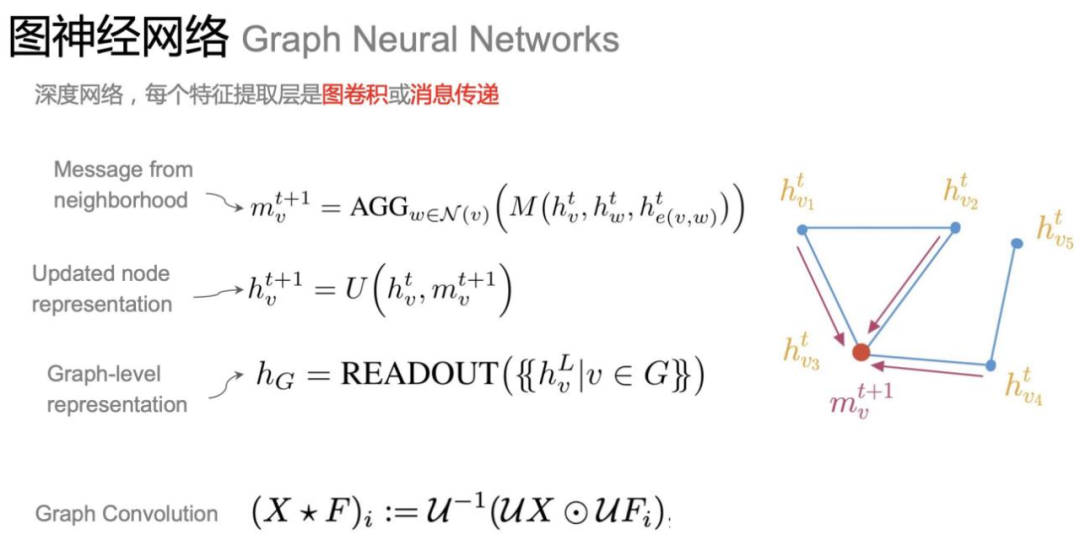25 0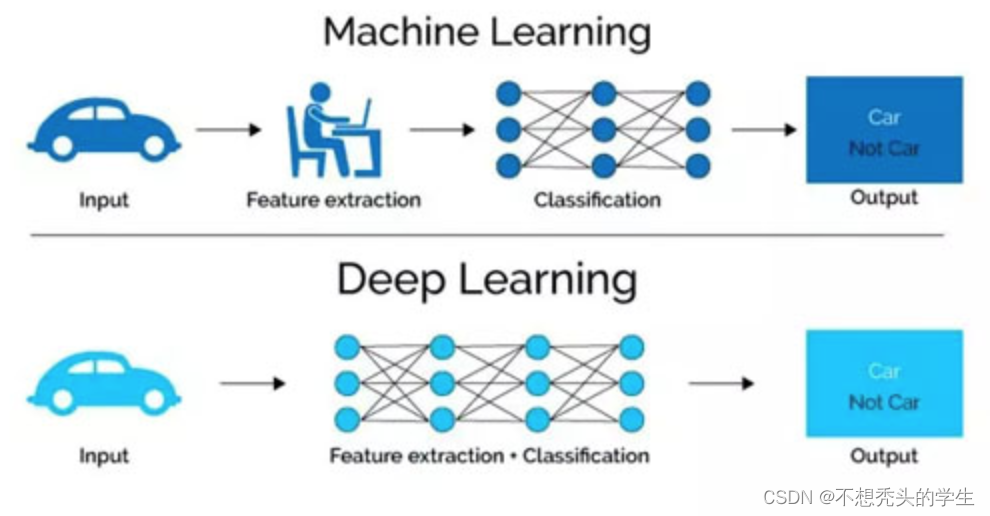25 0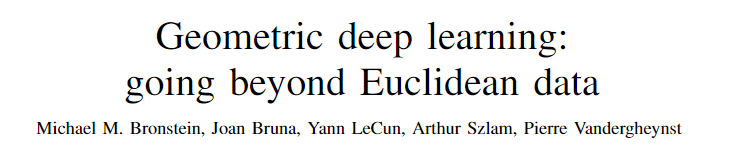「几何深度学习」从古希腊到AlphaFold，「图神经网络」起源于物理与化学
「几何深度学习」从古希腊到AlphaFold，「图神经网络」起源于物理与化学
15 0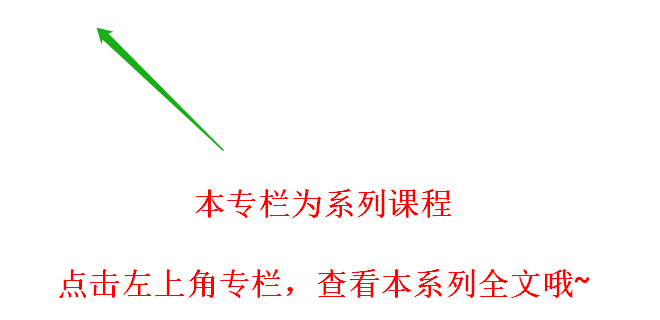【Pytorch神经网络基础理论篇】 01 从零开始介绍深度学习算法和代码实现

21 0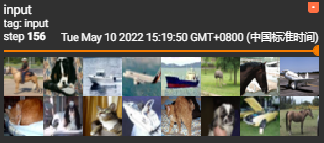【深度学习04】 快速构建一个神经网络

29 0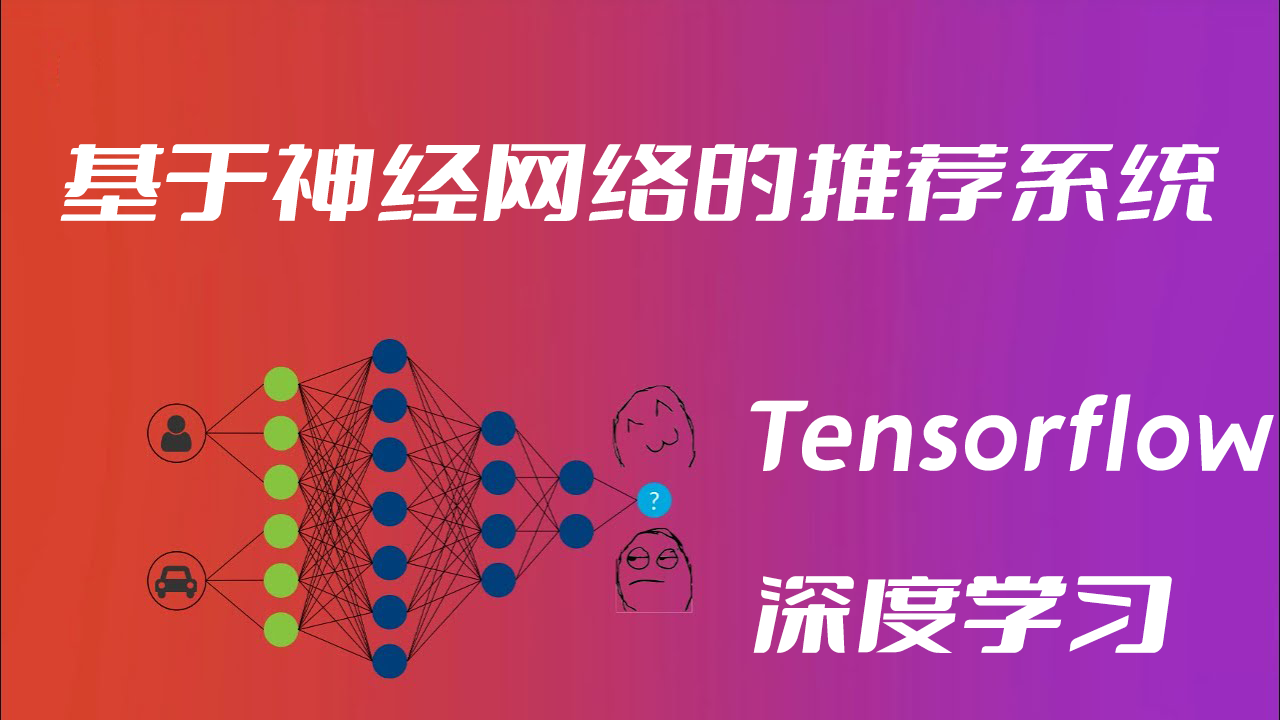63 0PyTorch 深度学习实战 |用 TensorFlow 训练神经网络

93 0PyTorch深度学习实战 | 神经网络的优化难题

89 045991